Statistical Approaches in Analysis of Variance: from Random Arrangements to Latin Square Experimental Design

Sorana D. BOLBOACĂ1,2,*, Lorentz JÄNTSCHI2,3, Radu E. SESTRAŞ2

1 “Iuliu Haţieganu” University of Medicine and Pharmacy Cluj-Napoca, Department of Medical Informatics and Biostatistics, 6 Louis Pasteur, 400349 Cluj-Napoca, Romania.

2 University of Agricultural Sciences and Veterinary Medicine Cluj-Napoca, 3-5 Mănăştur, 400372 Cluj, Romania.

3 Technical University of Cluj-Napoca, Department of Chemistry, 103-105 Muncii Bvd., 400641 Cluj-Napoca, Romania.

(* Corresponding author)

Abstract

Background: The choices of experimental design as well as of statistical analysis are of huge importance in field experiments. These are necessary to be correctly in order to obtain the best possible precision of the results. The random arrangements, randomized blocks and Latin square designs were reviewed and analyzed from the statistical perspective of error analysis. Material and Method: Random arrangements, randomized block and Latin squares experimental designs were used as field experiments. A series of previously published data were analyzed. An algorithm for errors analysis was developed and applied on the experimental data. Results: The analysis revealed that the errors classification in random arrangements is: Error(Treatment) < Error(Total) < Error(Experiment). The errors classification in randomized blocks revealed to be: Error(Treatment) < Error(Total) < Error(Experiment) < Error(Block). The obtained errors classification in Latin square was as follows: Error(Experiment) < Error(Treatment) < Error(Total) < Error(Column) < Error(Row). Conclusions: The Latin square design proved to have the smallest experimental errors compared to randomized arrangement and randomized block design. The classification of errors proved to be similar in randomized arrangements and randomized block design.

Keywords

Design of experiment; Random arrangements; Randomized blocks; Latin squares; Analysis of errors.

Introduction

The experimental design could be consider the golden state of any experiment due to its aims to ensure that the experiment is able to detect the treatment effects that are of interest by using the available resources to obtain the best possible precision. The choice of design as well as the choice of statistical analysis can make a huge difference. The methods of experimental design are obviously at least as important as method of data analysis.

First systematic experiment (a clinical trial) was developed and applied by James Lind in 1747 to treat the scurvy (deficiency of vitamin C) [1, 2]. Sir Ronald A. Fisher  was the fist statistician that applied a formal experimental mathematical model .

The peculiarity of field experiments lies in the fact, verified in all careful uniformity trials, that the area of ground chosen for the experimental plots may be assumed to be markedly heterogeneous, in that its fertility varies in a systematic, and often a complicated manner from point to point [4, 5]. This fact gives a risk to be in error when conclusions were drawn from a field experiment. Consequently, in order to be able to obtain statistically significant results from observations, the experiment should be designed in such manner in which the experiment settings (excepting the location for an observable) to be present more than once in the field (the confidence of the observation result increasing with the number of the locations of the observation). The direct way of overcoming this difficulty is to arrange the plots wholly at random.

The paper aims to present and analyze the error from the statistical point of view from random arrangements, randomized blocks and Latin square experimental designs.

Material and Method

First requirement of a well-designed experiment is that the experiment should yield a comparison of different manures, treatments, varieties and of testing the significance of such differences as are observed .

Randomized Design

The randomization is used in field experiments in order to avoid systematic, selection, accidental biases and to avoid the cheating by the experimenter.

The experimental data obtained by Mercer and Hall  in a uniformity trial on 20 plots and 5 treatments expressed as weights of mangold root were subject of random arrangements analysis. The following order was used in the experiment: B1 (3504), C1 (3430), A1 (3376), C2 (3334), E1 (3253), E2 (3314), E3 (3287), A2 (3361), D1 (3404), A3 (3366), B2 (3416), C3 (3291), B3 (3244), D2 (3210), D3 (3168), B4 (3195), A4 (3330), D4 (3118), C4 (3029), E4 (3085).

Randomized Block Design

The accuracy of the observation could be increased by using blocks (adding restrictions on the orders of strips arrangements). Thus, the analysis of randomized blocks was also performed. The variance is split three parts in randomized blocks design : ▪ Local differences between blocks; ▪ Differences due to treatment; ▪ Experimental errors. Thus, the estimate of experimental error becomes an unbiased estimate of the actual error in the differences due to treatment. A random arrangement (or systematic order) of in 4 blocks by imposing the condition that each treatment shall occur once in each block could lead to the following design:

§         Block 1: 3314 (A1) - 3416 (E1) - 3404 (C1) - 3029 (D1) - 3504 (B1)

§         Block 2: 3334 (C2) - 3207 (B2) - 3195 (E2) - 3287 (D2) - 3361 (A2)

§         Block 3: 3118 (A3) - 3085 (D3) - 3244 (E3) - 3167 (B3) - 3366 (C3)

§         Block 4: 3291 (C4) - 3330 (E4) - 3253 (B4) - 3376 (A4) - 3430 (D4)

The number of runs needed in complete randomized block designs  is directly related with the number of levels of factors implied in analysis. The general formula of number of runs is:

 nrun = Li × Li+1 × … × Lk Eq.1.

where nrun = number of runs; Li = number of levels of factor i (1 ≤ ik, k = number of factors). For example when we have 2 factors (treatment factor with five levels and blocking factor with 4 levels) with one replication per plot it will be needed a number of runs equal to L1 × L2 = 5 × 4 = 20 runs.

The following assumptions were done in error analysis in randomized blocks design (H0: the variation within block is the same with the variation within each treatment vs. Ha: the variation within block is not the same with the variation within each treatment or H0: the means of treatments are equal vs Ha: at least two means differ): ▪ the blocks are considered random; ▪ the measurement values follows a normal distribution with the same variance; ▪ the measurement errors are independent of the block effect; the block effects follows an identical normal distribution with a mean 0.

Latin Squares

Latin square design and the related Graeco-Latin square and Hyper-Graeco-Latin square designs are a special type of comparative design used to control the variation related to both rows and columns in the field experiment . These experimental designs are used when:

§         The analysis of several nuisance factors is desired and it is not proper to combine these factors into a single factor.

§         The research resources allows a relative small number of runs:

 Design Number of factors Number of runs 3×3 Latin square 3 9 3×3 Graeco-Latin Square 4 9 4×4 Latin square* 3 16 4×4 Graeco-Latin Square 4 16 4×4 Hyper-Graeco-Latin Square 5 16 * equivalent to a 43-1 fractional factorial design 

The design is limited to the experiments in which the number of levels of each blocking variable is equal with the number of levels of the treatment factor. Moreover, it could be applied under the assumption that there are no interactions between the blocking variables or between the treatment variable and the blocking variables. Note that the rows and columns are orthogonal to treatments in the Latin square design.

The general model of the response for a Latin square design is presented in Eq. 2.

 Yijk = μ + Ri + Cj + Tk + RE Eq.2.

where Yijk  = any observation for which X1 = i, X2 = j (X1, X2 = blocking factors), X3 = treatment factor; μ = general location parameter, Ri = effect for block i; Cj = effect for block j; Tk = effect for treatment; RE = random error (measure of the sum of variation between plots or units receiving same treatments).

The experimental data obtained by Mercer and Hall  in a block of 25 plots arranged in 5 rows and 5 columns, to be used for testing 5 treatments (each treatment occurs once in each row) was subject to Latin square analysis of errors (Table 1.).

Table 1. Experimental data: Latin square experimental design 

 Block 1 Block 2 Block 3 Block 4 Block 5 D 376 E 371 C 355 B 356 A 335 B 316 D 338 E 336 A 356 C 332 C 326 A 326 B 335 D 343 E 330 E 317 B 343 A 330 C 327 D 336 A 321 C 332 D 317 E 318 B 306

Results and Discussion

Randomized Design

If twenty observations are made at random for a single factor of primary interest for five values of the factor, then a random succession of the factor values must be assured such that exactly four repetitions of every value should be found in the succession.

The summary of the analysis of variance on the investigated sample is presented in Table 2.

The significance of numbers presented in Table 2 is as follows:

1.      Sum the observations on every treatment: give the total amount of the observable for a given treatment. Sum(A)=(A2+A1+A3+A4); Sum(B)=(B1+B2+B3+B4); Sum(C)=(C2+C1+C3+C4); Sum(D)=(D2+D1+D3+D4); Sum(E)=(E2+E1+E3+E4).

2.      Sum the sums to obtain the total amount of the observable for all treatments. Sum: SumT=Sum(A)+Sum(B)+Sum(C)+Sum(D)+Sum(E).

3.      Compute the average sum by treatment: AvgT=SumT/5.

4.      Compute the differences between the sums on treatment and average sum by treatment: Dif(A)=Sum(A)-AvgT; Dif(B)=Sum(B)-AvgT; Dif(C)=Sum(C)-AvgT; Dif(D)=Sum(D)-AvgT; Dif(E)=Sum(E)-AvgT.

Table 2. Analysis of variance in 5×4 random arrangement experiment

 Experimental A B C D E Treatment Observation 1 3376 3504 3430 3404 3253 Observation 2 3361 3416 3334 3210 3314 Observation 3 3366 3244 3291 3168 3287 Observation 4 3330 3195 3029 3118 3085 Sum (1 to 4)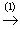13433 13359 13084 12900 12939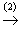Σ = 65715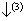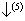Sum (1 to 4) - Σ/5 290 216 -59 -243 -204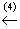Σ/5 = 13143 (Sum (1 to 4) - Σ/5)2 84100 46656 3481 59049 41616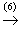Σ = 234902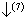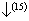SsqE = 289765.75 - 58725.5 = 231040.25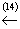StdD(1..4×A..E) = 123.49 Σ/4 = 58725.5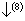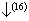MsqE = SsqE/(19-4) = 15402.68 MsqD(1..4×A..E) = 15250.83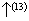Σ/4/4 = 14681.4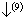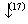StdE = √MsqE = 124.11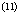SsqD(1..4×A..E) = 289765.75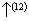√Σ/4/4 = 121.17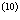degrees of freedom = 19-4 19 4 FT = 0.95 (p = 0.4628)

5.      Square the differences: Dif(A)2=(Sum(A)-AvgT)2; Dif(B)2=(Sum(B)-AvgT)2; Dif(C)2=(Sum(C)-AvgT)2; Dif(D)2=(Sum(D)-AvgT)2; Dif(E)2=(Sum(E)-AvgT)2.

6.      Sum the difference squared: SumDS = Dif(A)2+Dif(B)2+Dif(C)2+Dif(D)2+Dif(E)2.

7.      Compute the sum of squares (SsqT): SsqT=SumDS/4.

8.      Compute the mean of squares (MsqT): MsqT=SsqT/4.

9.      Compute the standard deviation (StdT) due to treatment: StdT=√MsqT.

10.  Compute the degree of freedom (dfT = degree of freedom for treatment; dfEE = degree of freedom for experimental error): dfT = number of treatments (5) - 1; dfEE = number of strips (20) – number of treatments (5).

11.  Compute the sum of squares for the total amount of data: SsqD=(A1-SumT/20)2+(A2-SumT/20)2+(A3-SumT/20)2+…+(C5-SumT/20)2.

12.  Compute the mean of squares for the total amount of data: MsqD = SsqD/(dfEE+dfT).

13.  Compute the standard deviation for the total amount of data: StdD = √MsqD.

14.  Compute the sum of squares of experimental errors: SsqE = SsqD-SsqT.

15.  Compute the mean of squares of experimental errors: MsqE=SsqE/(dfEE-dfT).

16.  Compute the standard deviation of the experimental errors: StdE=√MsqE.

17.  Test statistic for treatment: FT = MsqT/MsqE (follows F distribution with dfT and (dfT-dfT -1) degree of freedom). FT = 0.95 (p = 0.4628)

The analysis of the results presented in Table 3 revealed the following classification of errors (Eq. 3.):

 Error(Treatment) < Error(Total) < Error(Experiment) Eq.3.

The Eq. 3. is the common expected result under assumption that the treatment produces effects on the observable. Thus, an analysis of variance always should produce this relationship in regards of errors.

The analysis of variance for a randomized blocks design is presented in Table 3. The calculations were performed according to the formula used for Table 2.

Table 3. Analysis of Errors: randomized block design

 Treatment Block A B C D E SumB DifB DifB2 1 3314 (A1) 3504 (B1) 3404 (C1) 3029 (D1) 3416 (E1) 16667 238.25 56763.06 2 3361 (A2) 3207 (B2) 3334 (C2) 3287 (D2) 3195 (E2) 16384 -44.75 2002.56 3 3118 (A3) 3167 (B3) 3366 (C3) 3085 (D3) 3244 (E3) 15980 -448.75 201376.56 4 3376 (A4) 3253 (B4) 3291 (C4) 3430 (D4) 3330 (E4) 16680 251.25 63126.56 SumT 13169 13131 13395 12831 13185 65715 (SumTot) DifT 26 -12 252 -312 42 DifT2 676 144 63504 97344 1764 SumDif2 163432 (T) 323268.75 (B) Ssq 40858 (T) 107756.25 (B) 249607.75 (E) 290465.75 (Tot) Msq 10214.50 (T) 35918.75 (B) 20800.65 (E) 15287.67 (Tot) Std 101.07 (T) 189.52 (B) 144.22 (E) 123.64 (Tot) FT = 10214.50 / 20800.65 = 0.49 (p = 0.7428) FB = 35918.75 / 20800.65 = 1.73 (p = 0.2244) T = treatment; B = block number; E = Error; Tot = total amount of variation; SsqE = SsqTot – SsqT – SsqB; p = p-value; F = F-test; Test statistic for treatment: F dist with dfT (degree of freedom for treatment) and (number of observations – number of treatment – number of blocks -1) degree of freedom; Test statistic for effect of blocks: F dist with dfB (degree of freedom for blocks) and (number of observations – number of treatment – number of blocks -1) degree of freedom.

The analysis of the standard deviation resulted on randomized block design allows the following classification of errors (Table 3):

 Error(Treatment) < Error(Total) < Error(Experiment) < Error(Block) Eq.4.

The error produce by blocks proved to have the highest value, and it could be explained by the inappropriate analysis of the experimental data or breaking the test assumptions. This king of analysis is proper when the nuisance factor is known and controllable. If this factor is unknown and uncontrollable, the randomization could balance their impact across the experiment. The analysis of covariance is preferred if the nuisance factor is known but uncontrollable (the effect of nuisance factor is removed from the analysis). Note that several nuisance factors could combine in a block and influence the analysis of variance.

The test assumption on one-factor randomized block design is violated if [11, 12]: absence of independence between factor and blocking; existence of extreme values (outlier); non-normality of entire sample; patterns in plots of data; small sample size; and/or multiple comparison (more than one primary factor).

Latin Squares

The results obtained in analysis obtained in investigation of Latin square experimental design are presented in Table 4.

Table 4. Error analysis: Latin square design

 1 2 3 4 5 SumR DifR DifR2 1 376 371 355 356 335 1793 117.4 13782.76 2 316 338 336 356 332 1678 2.4 5.76 3 326 326 335 343 330 1660 -15.6 243.36 4 317 343 330 327 336 1653 -22.6 510.76 5 321 332 317 318 306 1594 -81.6 6658.56 SumC 1656 1710 1673 1700 1639 8378 (SumTot) DifC -19.6 34.4 -2.6 24.4 -36.6 DifC2 384.16 1183.36 6.76 595.36 1339.56 SumDif2 3509.2 (C) 21201.2 (R) 1651.2 (T) Ssq 877.3 (C) 5300.3 (R) 412.8 (T) 436.24 (E) 7026.64 (Tot) Msq 219.3 (C) 1325.1 (R) 103.2 (T) 36.4 (E) 292.78 (Tot) Std 14.8 (C) 36.4 (R) 10.2 (T) 6.0 (E) 17.1 (Tot) FT = 103.2 / 36.4 = 2.84 (p = 0.0618) C = column; R = row; T = treatment; E = error; Tot = total

The analysis on Latin square design allows the following classification of errors (Table 4):

 Error(Experiment) < Error(Treatment) < Error(Total) < Error(Column) < Error(Row) Eq.5.

The analysis of error classification according with the investigate designs of experiments revealed the following:

§         The randomized arrangements and randomized block design produce the same classification of errors.

§         The smallest error proved to be of treatment in randomized arrangement and randomize block design and of experiment in Latin square.

§         The error of experiment proved to the have the smaller value in Latin square design but the highest value in randomized blocks and randomized arrangements.

There are a lot of programs which allows the analysis of error and variance according ort not to experimental design [13-18]. A series of designs also were developed and applied in order to reduce different kind of errors and to respond to different restrictions: independent replications and the same square used in replications ; semi-Latin squares [20, 21]; split plot experimental design ; clustered versus spatial randomized blocks ; non-randomized block design . New statistical techniques were proposed to be used for analysis of data resulted from randomized block designs and Latin squares: Sign and Hotelling-Hsu's T2 tests ; Friedman and Page tests ; rank test for a randomized block design based on the quantile score used when a small proportion of the observations from each treatment group shows very high response values or when the response is dichotomous ; locally most powerful (LMP) rank test . In these situations, the researchers must have wide knowledge in at least 2 domains in order to be able to conduct a correct experiment: design of experiment and statistical analysis.

The field experiments should be correctly designed and analyzed in order to provide the maximum amount of information for the minimum amount of resources. The main criteria for a well-designed experiment recommended to be carefully addressed are:

1.      Unbiased (identical as possible environmental conditions): allows an accurate and valid comparison between treatment groups.

2.      High precision (uniform selection of experimental material; increasing the number of observations): allows identifying the true effect is any exists.

3.      Wide target of applicability: allows exploration of other nuisance factors.

4.      Simple experiments (as simple as possible).

Last but not the least, a well-design experiment should provide all data necessary to calculate uncertainty (capable to be statistically analyzed and to quantify the level of confidence in the results) without which the experiment is useless.

Conclusions

A correct experimental design is as important as a correct statistical analysis in order to obtain valid and reliable conclusion from field experiments. Certain restrictions must be imposed when the plots are arranged in order to be able to accurately estimate the errors.

The Latin square design proved to have the smallest experimental error compared to randomized arrangement and randomized block design. The classification of errors proved to be similar in randomized arrangements and randomized block design.

References

1.            Dunn P., James Lind (1716-94) of Edinburgh and the treatment of scurvy, Archive of Disease in Childhood Foetal Neonatal 1997, 76, p. 64-65.

2.            Carlisle R., Scientific American Inventions and Discoveries, John Wiley & Songs, Inc., New Jersey, 2004.

3.            Fisher R. A., The Principles of Experimentation, Illustrated by a Phycho-Physical Experiment. In: Fisher R. A. (Ed) The Design of Experiments, 9th Edition. Hafner Publishing Company; New York, 1971.

4.            Fisher R. A., Statistical Methods for Research Workers, Edinburgh: Oliver and Boyd, 1925.

5.            Fisher R. A., The arrangement of field experiments, Journal of Ministry of Agriculture, 1926, 33, p. 503-513.

6.            Mercer W. B., Hall A. D., The experimental error of field trials, Journal of Agricultural Science 1911, IV, p. 107-132.

7.            Hinkelmann K., Kempthorne O., Design and Analysis of Experiments. I and II (Second ed.). Wiley, 2008.

8.            Shieh G., Jan S.-L., The effectiveness of randomized complete block design, Statistica Neerlandica 2004, 58(1), p. 111-124.

9.            Cochran W. G., Early development of techniques in comparative experimentation, On the History of Statistics and Probability, 1976, pp. 3-25.

10.        Box G. E. P., Hunter W. G., Hunter S. J., Statistics for Experimenters, John Wiley & Sons, Inc., New York, NY, 1978.

11.        Searle S. R., Casella G., McCulloch C., Variance Components, New York: Wiley, 1992.

12.        Sokal R. R., Rohlf F. J., Biometry: The Principles and Practice of Statistics in Biological Research, Second edition. New York: W. H. Freeman and Company, 1981.

13.        Tjur T., An algorithm for optimization of block designs. Journal of Statistical Planning and Inference, 1993, 36(2-3), p. 277-282.

14.        De Assis Santos E Silva F., De Azevedo C. A. V., A new version of the assistant-statistical assistance software, Computers in Agriculture and Natural Resources - Proceedings of the 4th World Congress, 2006, pp. 393-396.

15.        Li L.-F., Dai Y., Zhang G.-Q., Generating test-case based on orthogonal latin squares, Journal of Soochow University Engineering Science Edition, 2006, 26(2), pp. 19.

16.        Anu S., Cini V., Rao A. R., Gupta V. K., Soumen P., SPRMD-A statistical package for cataloguing and generation of repeated measurements designs, Journal of Indian Society of Agricultural Statistics, 2007, 61(1), 84-90.

17.        Gligoroski D., Markovski S., Knapskog S. J., The stream cipher Edon80, Lectures Notes in Computer Science, 2008, 4986, p. 152-169.

18.        Sharma A., Varghese C., Rao A. R., Software for the analysis of repeated measurements designs, Indian Journal of Animal Sciences, 2009, 79(4), 445-448.

19.        Edwards A. L., Homogeneity of variance and the Latin square design, Psychological Bulletin, 1950, 47(2), p. 118-129.

20.        Bailey R. A., Semi-Latin squares, Journal of Statistical Planning and Inference, 1988, 18(3), p. 299-312.

21.        Bailey R. A., An efficient semi-latin square for twelve treatments in blocks of size two, Journal of Statistical Planning and Inference, 1990, 26(3), p. 263-266.

22.        Federer W. T., Arguillas Jr. F. O., Augmented split plot experiment design, Journal of Crop Improvement, 2005, 15(1), p. 81-96.

23.        Hoosbeek M. R., Stein A., van Reuler H., Janssen B. H., Interpolation of agronomic data from plot to field scale: using a clustered versus a spatially randomized block design, Geoderma, 1998, 81(3-4), p. 265-280.

24.        Bhaumik D. K., Mathew T., On the minimax optimality of non-randomized block designs, Computational Statistics & Data Analysis, 1989, 8(1), p. 59-66.

25.        Mahfouda Z. R., Randles R. H., Practical tests for randomized complete block designs, Journal of Multivariate Analysis, 2005, 96(1), p. 73-92.

26.        Möttönen J., Hüsler J., Oja H., Multivariate nonparametric tests in a randomized complete block design, Journal of Multivariate Analysis, 2003, 85(1), p. 106-129.

27.        Sim S., Kim S., Park G., Park J., A two sample quantile test with applications to randomized block designs, Statistics & Probability Letters, 2003, 61(4), p. 439-445.

28.        Nugroho S., Govindarajulu Z., Nonparametric tests for random effects in the balanced incomplete block design, Statistics & Probability Letters, 2002, 56(4), p. 431-437.